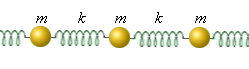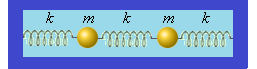## Monday, July 19, 2021

### Waves in Medium: UNIZOR.COM - Physics4Teens - Waves - Waves in Medium

Notes to a video lecture on http://www.unizor.com

Waves in Medium

This lecture is about a different type of waves.
These waves are still mechanical oscillations in a sense that they are movements of objects with some periodicity, but the objects are not exactly the same as we studied in a previous chapter "Mechanical Oscillations".
The objects we discuss in this lecture are molecules of some substance, like air or water, or steel.

The word medium we use in a sense of "a substance regarded as the means of transmission of a force or effect", as defined in Merriam-Webster dictionary. Examples are air, water, wood, glass etc.
Basically, any substance can be a medium and transmit an oscillation from its source to some destination. The only thing that cannot be a medium in this sense is an absolute vacuum void of any force fields.

As an example of oscillation of a medium, consider a ringing bell after we strike it with a hammer.
For quite some time molecules inside this bell are oscillating, forcing to oscillate air around a bell as well, so we hear the sound.

Let's create a simplified model of this process.
First of all, we will research only a one-dimensional case.
As an example, consider a propagation of oscillation inside a thin metal rod after we strike one of its ends with a hammer.

Further consider that each molecule of a metal this thin rod is made of is connected by spring-like inter-molecular links with its left and right neighbors along a rod. If one molecule is oscillates, it will squeeze one link that attaches it to one neighboring molecule and stretches the other link, thus engaging its neighbors in oscillation.
In our one-dimensional case this model looks like thiswhere letter m indicates a molecule with its mass, while letter k indicates an elasticity of a weightless spring-like connection between molecules.

The above model of propagation of oscillation, while not perfect, allows to better understand the mechanism of waves in medium.

Finally, we simplify the problem even further by limiting the length of a rod to only two molecules, thus making the following model.In this model two identical molecules, #1 (left on the picture) and #2 (right), of mass m each are linked by identical weightless springs of length L and elasticity k among themselves and to the edges of a thin rod.
Assume that initially the left end of the rod is at the origin of X-axis, the molecule #1 is at distance L from it along the X-axis, the molecule #2 is at distance L from the first further along the X-axis and the right end of a rod is by L further down. This assumption means that initially all springs are in neutral position.
Also assume that the molecule #1 (left on the picture above) has an initial speed v, modeling a strike of a hammer on the bell.

Let x1(t) be a displacement of the molecule #1 (left on the picture above) from its initial position at moment in time t and x2(t) be a displacement of the molecule #2 (right on the picture) from its initial neutral position at time t.
Assume, the initial displacement of molecules at time t=0 is:
x1(0)=0
x2(0)=0
Their initial velocity is:
x'1(0)=v
x'2(0)=0

There are two forces acting on the molecule #1 (left on the picture above) from two springs on both sides of it, which are:
F1L(t) by the left spring attached to the left edge and
F12(t) by the middle spring attached to the molecule #2.
There are two forces acting on the molecule #2 (right) from two springs on both sides of it, which are:
F2R(t) by the right spring attached to the right edge and
F21(t) by the middle spring attached to the molecule #1.

The direction (sign) of these forces depends on the displacement of molecules, we will take it into account when constructing the equations based on the Hooke's Law.
Also, forces F12(t) by the middle spring on the first molecule and F21(t) by the middle spring on the second molecule are opposite in direction and equal by absolute value since they depend only on the length of the middle spring.

The resulting force acting on the molecule #1 is
F1(t) = F1L(t) + F12(t)
The resulting force acting on the molecule #2 is
F2(t) = F2R(t) + F21(t)

If the displacements of molecules #1 (left on the picture above) and #2 (right) at any moment of time t are, correspondingly, x1(t) and x2(t), the forces F1L(t) of the left spring and F2R(t) of the right spring, acting on molecules #1 and #2 correspondingly, according to the Hooke's Law, are
F1L(t) = −k·x1(t)
F2R(t) = −k·x2(t)

The forces of the middle spring acting on molecules #1 and #2 depend on the difference in displacements of these molecules from their neutral position.

Let
Δ(t) = x1(t) − x2(t)

If Δ(t) is positive, the middle spring is squeezed and its left end (towards molecule #1) pushes molecule #1 to the left (in negative direction), which makes F12(t) negative, while its right end pushes to the right (in positive direction) molecule #2, which makes F21(t) positive.

If Δ(t) is negative, the middle spring is stretched and its left end (towards molecule #1) pulls molecule #1 to the right (in positive direction), which makes F12(t) positive, while its right end pulls to the left (in negative direction) molecule #2, making F21(t) negative.

Therefore,
F12(t) = −k·Δ(t)
F21(t) = k·Δ(t)

Now we can express the resulting force acting on the molecule #1 is
F1(t) = −k·x1(t) − k·Δ(t) =
= −k·
[x1(t) + x1(t) − x2(t)]
And the resulting force acting on the molecule #2 is
F2(t) = −k·x2(t) + k·Δ(t) =
= −k·
[x2(t) − x1(t) + x2(t)]

Taking into account the Second Newton's Law, F1(t)=m·x"1(t) and F2(t)=m·x"2(t), we obtain the following system of two linear homogeneous differential equations of second order
m·x"1(t) = −2k·x1(t) + k·x2(t)
m·x"2(t) = k·x1(t) − 2k·x2(t)
Bringing all members to the left part of equations, we get m·x"1(t) + 2k·x1(t) − k·x2(t) = 0
m·x"2(t) − k·x1(t) + 2k·x2(t) = 0
or, using the familiar concept of angular speed ω²=k/m,
x"1(t) + 2ω²·x1(t) − ω²·x2(t) = 0
x"2(t) − ω²·x1(t) + 2ω²·x2(t) = 0

Let's approach this system of equations in a more "mathematical" way, using vectors and matrices (you can refer to "Math 4 Teens" course on UNIZOR.COM, which explains the concepts of a vector and a matrix and operations with them).

Consider a vector X(t) of displacements
 x1(t) x2(t)
and a matrix Ω of coefficients
 2ω² −ω² −ω² 2ω²

Now our system of two differential equations can be represented as one equation with vector argument
X"(t) + Ω·X(t) = 0
where X"(t) signifies a vector of second derivatives of each component of a vector X(t) and 0 signifies a null-vector.
An operation · (dot) in this equation signifies a multiplication of a matrix Ω by a vector X(t).

Compare it with an equation for a periodic movement we discussed in the very first lecture on Waves
x"(t) + (k/m)·x(t) = 0
where later on we assigned ω²=k/m.
We will try to solve a vector equation the same way we solved this similar equation in a one-dimensional case.
This is a subject of the next lecture.